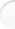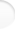Structure of Magic and Semi-Magic Squares, Methods and Tools for Enumeration by Francis GaspalouThis page deals with transformations on the contents of the cells (and not on their position as in the previous page).

 1. Principle of the Method

The method consists in deducting from one square another square by a permutation on the n² first numbers: each number is substituted for its exchanged. The transformation is on the contents of cells and not on their position.
If the transformed square is magic (or semi magic), it is said that the permutation is applied to such a square.

Two squares are sufficient to define a permutation: it is the permutation which allows to pass from the first to the second, that is to say which makes a correspondence between the contents of each cell of the first square and the same cell of the second square.

Ex 1: the permutation which makes a correspondence between each number x and n²+1-x (complement to n²+1) can be applied to each magic square or semi-magic square of order n. Therefore, the number of magic squares or semi-magic squares of order n is even.

Ex 2: 4 specific permutations can be applied to each semi-regular squares (see definition in the next page, The intermediate square method, § 4):
• capital letter + lower-case letter (permutation identity)
• capital letter + complement to m for lower-case letter,
• complement to M for capital letter + lower-case letter,
• complement to M for capital letter + complement to m for lower-case letter (it is "permutation complement to n²+1" of Ex 1).
M is the sum of extremes for capital letters, m for lower-case letters and M+m=n²+1 (see next page).
By permuting capital and lower-case letters - which is possible only for a part of these semi-regular squares - 4 other supplementary permutations are obtained; therefore a total of 8 permutations for this part of semi-regular squares. In fact, this group of order 8 is only a subgroup of a larger group of permutations: the group on regular squares.

Ex 3: the permutation by 2 modulo 17 on magic squares of order 4. You find a set of 5 696 squares among the total of 7 040 squares of order 4.

The image of a permutation is the classical image of a permutation on the n² first numbers. For example, for the permutation complement to n²+1 and for n=4, it is the permutation:
 ↓ 1 2 3 ... 16 16 15 14 ... 1
or in a reduced form:
 ↓ 16 15 14 ... 1

Note that the intermediate square method (see next page) constitutes a special case of the permutation method (it is a special permutation obtained with a particular configuration of capital letters and lower-case letters; magic conditions are on capital and lower-case letters, that is to say on the contents of cells).

 2. Properties of Permutations Top of the pageTo each permutation, it can be associated a set of squares which is the space of definition of the permutation on the given field.

Permutations can be composed: you find the classical product of two permutations.

Between two given squares, a transformation and a permutation can be defined to pass from the first to the second. These two operators have the same order. I have no demonstration of this property.

 3. Results Top of the pageThe internal permutations to a set constitutes a group G'. But, besides the permutation identity, only one permutation was found on the field of magic squares (or semi-magic squares): the permutation "complement to n²+1". That is to say the group G' (permutations) is only of order 2 for all the squares.

Note: the permutation "complement to n²+1" is the permutation which makes a correspondence between each number and its complement to n²+1. Therefore, this permutation is also the transformation "diagram" which represents the "type" of the square.

But you can have sets of squares with group G' (permutations) of order larger than 2.

On a same set of squares, you find a group G, of a certain order, for the transformations and another group G', of a different order, for the permutations. Generally, there is not equality (there is equality if the set of squares has a group structure: I haven't a general demonstration, but it is true probably true).

E.g.:
SetOrder of group G
(transformations)
Order of group G'
(permutations)
7 040 magic squares of order 4322
3 456 semi-pandiagonal1 152384
384 pandiagonal squares384384

The transformation and permutation methods can be combined. But you have to be very careful when calculating the number of elementary squares: an induced square by transformation can be identical to an induced square by permutation! Consequently, the number of induced squares - both by transformations and by permutations - is not the same for all the elementary squares.
• For example, on the 7 040 magic squares of order 4, the 220 elementary squares obtained with transformation method are reduced to 166 if the permutation method is then applied after:  112 squares with 32 induced squares (transformation * permutation)= 3 584 squares 54 squares with 64 induced squares (transformation * permutation)= 3 456 squares 166 7 040
166 is the lower number of elementary squares you can have (with operation good for all the 7 040 squares of the set; you exclude operation specific to subsets). This result seems original.
I identified all these squares. The 3 584 squares are of DUDENEY's types I, II, III and VI. The 3 456 squares are of DUDENEY's remaining types: IV, V, VII to XII (these 3 456 squares are different from the 3 456 semi-pandiagonal squares).
For the 112 elementary squares, the permutation "complement to 17" is identical to a transformation of group G of 32: you have only 32 induced squares. For the 54 elementary squares, this permutation gives a new square and you have 64 induced squares.
• For magic squares of order 5, you can reduce enumeration to squares with centre running from 1 to 13 with the permutation "complement to 26" (and use the transformation method for the different A1).

Note: the two combined methods (transformation and permutation) can also be applied to enumeration of lower-case letter squares (e.g.: Latin squares or Gallic squares). See for vocabulary the next page, The intermediate square method, § 4. Then, for permutation method, the permutations are with the numbers 1 to n (and not 1 to n²). Examples of application are given in Appendix 3, § 5 for Gallic squares, in Appendix 4, § 4 and Appendix 5, § 1 for Latin diagonal squares.